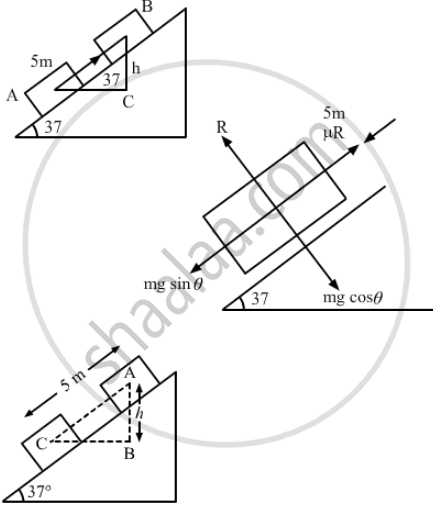Advertisement Remove all ads

# Question is Missing - Physics

Sum

A block of mass 2.0 kg is pushed down an inclined plane of inclination 37° with a force of 20 N acting parallel to the incline. It is found that the block moves on the incline with an acceleration of 10 m/s2. If the block started from rest, find the work done (a) by the applied force in the first second, (b) by the weight of the block in the first second and (c) by the frictional force acting on the block in the first second. Take g = 10 m/s2.

Advertisement Remove all ads

#### Solution

Given:

$\text{ Mass, m = 2 kg }$

$\text{ Inclination, } \theta = 37^\circ$

$\text{ Force applied, F = 20 N }$

$\text{ Acceleration of the block, a = 10 m/ s}^2$

(a) t = 1 sec

So,

$s = \text{ ut } + \frac{1}{2}\text{ at }^2 = 5 \text{m }$Work done by the applied force,

$\text{ W = Fs } \cos \theta^\circ= 20 \times 5 = 100 J$
(b) $AB \left( \text{ h } \right) = 5 \sin 37^\circ= 3 \text{ m }$
So, work done by weight,

$\text{ W = mgh}$

$2 \times 10 \times 3 = 60 J$

So, frictional force,

$\text{ f = mg } \sin \theta$
Work done by the friction forces,

$\text{ W = fs } \cos 0^\circ= \left( \text{ mg } \sin \theta \right) s$

$= 20 \times 0 . 60 \times 5 = - 60 J$

Is there an error in this question or solution?
Advertisement Remove all ads

#### APPEARS IN

HC Verma Class 11, 12 Concepts of Physics 1
Chapter 8 Work and Energy
Q 17 | Page 133
Advertisement Remove all ads
Advertisement Remove all ads
Share
Notifications

View all notifications

Forgot password?